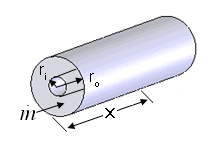Contents - Index

AnnularFlow_localProcedure AnnularFlow_local( Fluid\$, T, P, m_dot, r_i, r_o, L, RelRough: h_T_x, h_H_x, dPdx) returns lower and upper bounds for the local convection coefficient and the local pressure gradient for flow in an annular duct.  The local values are determined at axial position x by numerically differentiating the average values.  The outer wall of the annulus is assumed to be insulated so heat transfer occurs across the wall separating the annulus from the pipe.  The area used for heat transfer should be the area of this wall.

Inputs:

Fluid\$ can be any fluid in the EES database.  The fluid can be an ideal gas or a real fluid.

T - the bulk temperature of the fluid [C], [K], [F], or [R].

P -  pressure can be in [Pa], [kPa], [bar], [MPa], [atm], or [psia].

m_dot  -  mass flow rate in [kg/s] or [lbm/hr]

r_i - inner radius of annulus [m] or [ft]

r_o - outer radius of annulus [m] or [ft]

x - location along the flow axis [m] or [ft]

RelRough - the ratio of the dispersions on the walls of the duct to the hydraulic diameter  of the duct (must be between 0 and 0.05).  The hydraulic diameter is 2 W H/(W + H).

Outputs: (all but the first output are optional)

h_T_x -  lower bound on the local heat transfer coefficient in [W/m^2-K] or [Btu/hr-ft^2-R] assuming that the inner annulus wall is at constant temperature

h_H_x -  upper bound on the local heat transfer coefficient in [W/m^2-K] or [Btu/hr-ft^2-R] assuming a constant heat flux at the inner annulus wall

dPdx -  local pressure gradient.  [(Pa, kPa, bar,  or atm)/m] or  [(psi or atm)/ft] depending on EES pressure unit setting

Notes:

This procedure determines the local values by differentiating the average values calculated in the AnnularFlow procedure  .

Two values of the heat transfer coefficient are returned that provide upper and lower bounds.  These heat transfer coefficients are both for the inner annular wall with an insulated outer wall.  For turbulent flow, these values are identical.

Example:

\$unitSystem SI K Pa J

T=363 [K]   "average temperature of fluid in duct"

P=101300 [Pa]   "pressure of air in annulus"

m_dot=0.001 [kg/s]   "flow rate"

x=5 [m]    "length"

RelRough=0.005 [-] "relative roughness"

call AnnularFlow_local('air', T, P, m_dot, r_i, r_o, x, RelRough:h_T_x, h_H_x, dPdx)

{Solution:

dPdx=-0.704 [Pa/m]

h_H_x=6.598 [W/m^2-K]

h_T_x=6.205 [W/m^2-K]}